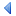UpTOC Index << 7 Included Tools < 8.28 Euphoria Database (EDS) Up: 8 API Reference 8.30 Flags > 9 Release Notes >>

## 8.29 Prime Numbers

### 8.29.1 Routines

#### 8.29.1.1 calc_primes

```include std/primes.e
namespace primes
public function calc_primes(integer approx_limit, atom time_limit_p = 10)
```

Returns all the prime numbers below a threshold, with a cap on computation time.

##### Parameters:
1. approx_limit : an integer, This is not the upper limit but the last prime returned is the next prime after or on this value.
2. time_out_p : an atom, the maximum number of seconds that this function can run for. The default is 10 (ten) seconds.
##### Returns:

A sequence, made of prime numbers in increasing order. The last value is the next prime number that falls on or after the value of approx_limit.

• The approx_limit argument does not represent the largest value to return. The largest value returned will be the next prime number on or after
• The returned sequence contains all the prime numbers less than its last element.
• If the function times out, it may not hold all primes below approx_limit, but only the largest ones will be absent. If the last element returned is less than approx_limit then the function timed out.
• To disable the timeout, simply give it a negative value.
##### Example 1:
```? calc_primes(1000, 5)
-- On a very slow computer, you may only get all primes up to say 719.
-- On a faster computer, the last element printed out will be 1009.
-- This call will never take longer than 5 seconds.
```

#### 8.29.1.2 next_prime

```include std/primes.e
namespace primes
public function next_prime(integer n, object fail_signal_p = - 1, atom time_out_p = 1)
```

Return the next prime number on or after the supplied number

##### Parameters:
1. n : an integer, the starting point for the search
2. fail_signal_p : an integer, used to signal error. Defaults to -1.
##### Returns:

An integer, which is prime only if it took less than 1 second to determine the next prime greater or equal to n.

The default value of -1 will alert you about an invalid returned value, since a prime not less than n is expected. However, you can pass another value for this parameter.

##### Example 1:
```? next_prime(997)
-- On a very slow computer, you might get -997, but 1009 is expected.
```

#### 8.29.1.3 prime_list

```include std/primes.e
namespace primes
public function prime_list(integer top_prime_p = 0)
```

Returns a list of prime numbers.

##### Parameters:
1. top_prime_p : The list will end with the prime less than or equal to this value. If top_prime_p is zero, the current list of calculated primes is returned.
##### Returns:

An sequence, a list of prime numbers from 2 to <= top_prime_p

##### Example 1:
```sequence pList = prime_list(1000)
-- pList will now contain all the primes from 2 up to the largest less than or
--    equal to 1000, which is 997.
```UpTOC Index << 7 Included Tools < 8.28 Euphoria Database (EDS) Up: 8 API Reference 8.30 Flags > 9 Release Notes >>